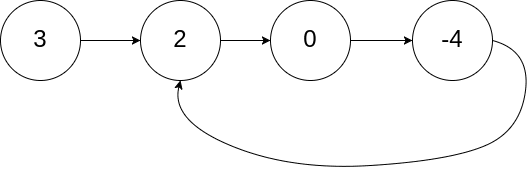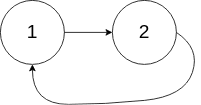# Question

Formatted question description: https://leetcode.ca/all/141.html

Given head, the head of a linked list, determine if the linked list has a cycle in it.

There is a cycle in a linked list if there is some node in the list that can be reached again by continuously following the next pointer. Internally, pos is used to denote the index of the node that tail's next pointer is connected to. Note that pos is not passed as a parameter.

Return true if there is a cycle in the linked list. Otherwise, return false.

Example 1:Input: head = [3,2,0,-4], pos = 1
Output: true
Explanation: There is a cycle in the linked list, where the tail connects to the 1st node (0-indexed).


Example 2:Input: head = [1,2], pos = 0
Output: true
Explanation: There is a cycle in the linked list, where the tail connects to the 0th node.


Example 3:Input: head = , pos = -1
Output: false
Explanation: There is no cycle in the linked list.


Constraints:

• The number of the nodes in the list is in the range [0, 104].
• -105 <= Node.val <= 105
• pos is -1 or a valid index in the linked-list.

Follow up: Can you solve it using O(1) (i.e. constant) memory?

# Algorithm

Two pointers, a slow pointer that walks one step at a time and a fast pointer that walks two steps at a time. If there is a ring in the linked list, the two pointers will definitely meet at the same position eventually.

Or, use a set for duplicate check.

# Code

• import java.util.HashSet;
import java.util.Set;

/**
* class ListNode {
*     int val;
*     ListNode next;
*     ListNode(int x) {
*         val = x;
*         next = null;
*     }
* }
*/
public class Solution {
return false;
}

while (fast != null && fast.next != null) {
slow = slow.next;
fast = fast.next.next;
if (slow == fast) return true;
}

return false;
}
}

public class Solution_extra_space {

Set<ListNode> hs = new HashSet<ListNode>();

while (current != null) {

if (hs.contains(current)) {
return true;
}

current = current.next;
}

return false;

}
}

}

############

/**
* class ListNode {
*     int val;
*     ListNode next;
*     ListNode(int x) {
*         val = x;
*         next = null;
*     }
* }
*/
public class Solution {
while (fast != null && fast.next != null) {
slow = slow.next;
fast = fast.next.next;
if (slow == fast) {
return true;
}
}
return false;
}
}

• // OJ: https://leetcode.com/problems/linked-list-cycle/
// Time: O(N)
// Space: O(1)
class Solution {
public:
while (q && q->next) {
p = p->next;
q = q->next->next;
if (p == q) return true;
}
return false;
}
};

• # Definition for singly-linked list.
# class ListNode:
#     def __init__(self, x):
#         self.val = x
#         self.next = None

class Solution:
def hasCycle(self, head: ListNode) -> bool:
while fast and fast.next:
slow, fast = slow.next, fast.next.next
if slow == fast:
return True
return False

############

# class ListNode(object):
#     def __init__(self, x):
#         self.val = x
#         self.next = None

class Solution(object):
"""
:rtype: bool
"""
while fast and fast.next:
fast = fast.next.next
slow = slow.next
if slow == fast:
return True
return False


• /**
* type ListNode struct {
*     Val int
*     Next *ListNode
* }
*/
for fast != nil && fast.Next != nil {
slow, fast = slow.Next, fast.Next.Next
if slow == fast {
return true
}
}
return false
}

• /**
* class ListNode {
*     val: number
*     next: ListNode | null
*     constructor(val?: number, next?: ListNode | null) {
*         this.val = (val===undefined ? 0 : val)
*         this.next = (next===undefined ? null : next)
*     }
* }
*/

function hasCycle(head: ListNode | null): boolean {
const set = new Set<ListNode>();
while (node != null) {
if (set.has(node)) {
return true;
}
node = node.next;
}
return false;
}


• /**
* function ListNode(val) {
*     this.val = val;
*     this.next = null;
* }
*/

/**
* @return {boolean}
*/
var hasCycle = function (head) {
while (fast && fast.next) {
slow = slow.next;
fast = fast.next.next;
if (slow == fast) {
return true;
}
}
return false;
};